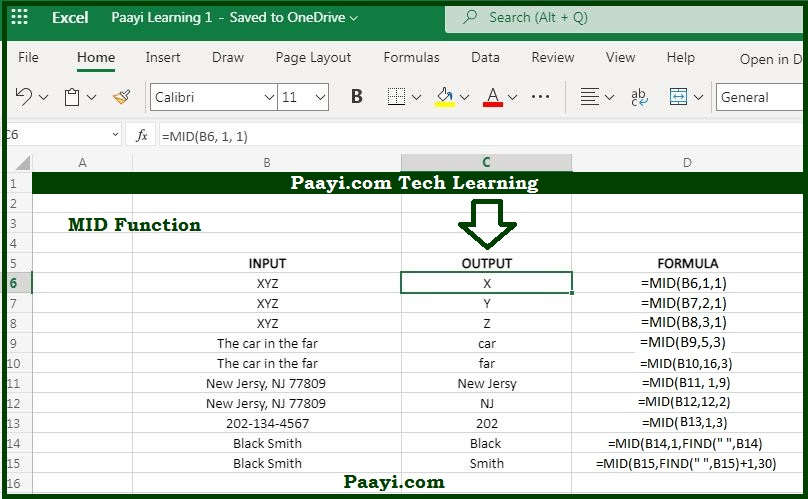# Learn How to Use Microsoft Excel MID Function

Written by | 0 Comments | 791 Views

In this article, you will learn how to use the Microsoft Excel MID function and its prime function in Microsoft Excel. You will also get to know the Microsoft Excel MID function return value and syntax with the help of some examples.

## Microsoft Excel MID Function

The main objective of the MID function is to extract text from the middle of the text. That means the Microsoft Excel MID function extracts a given number of characters from the middle of the text string provided. Let us understand this by the example:

= MID("Angle",2, 3) will return "ngl".

### MID Function Return Value

It will return the value as characters extracted.

### MID Function Syntax

= MID(text, start-num, num-chars)

Where the arguments:

• text: This is the text to extract from.
• start-num: The location of the first character to extract.
• num-chars: The number of characters to be extracted.

## How to Use Microsoft Excel MID Function?As we know, the MID function is used to extract the number of characters from the middle of the text string provided. The arguments - text, start-num, and num-chars are used with the MID function. In case num-chars is greater than the number of characters available, then the MID function will return as many characters as possible.

You can also combine the MID function with other functions like - LEN and FIND function to perform more complex operations. Let us understand the MID function with the help of examples given below:

Example 1: = MID("The car in the far" ,5, 3) - The return value will be "car".

Example 2: =MID("The car in the far", 16, 3) - The return value will be "far".